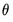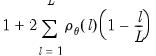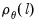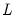makeif
Outputs inefficiency factors of the draws of the parameters in a BTVCVAR model.
Generates three matrix objects (one per parameter block) containing inefficiency factors. The inefficiency factor of the draws ofis defined aswhereis the sample autocorrelation in the draws ofat lag, andis the lag at which the sample autocorrelation tapers off. Values near one are desirable. Inefficiency factors much greater than one indicate slow mixing. An NA is returned if sample autocorrelation does not taper off sufficiently quickly (i.e., if sample autocorrelation does not fall below 0.05 in the first 100 lags).
The inefficiency factor can be interpreted as the ratio of the numerical variance of the sample mean computed using MCMC draws and the numerical variance of the sample mean computed using hypothetical IID draws. It measures the extent of efficiency loss for using MCMC instead of vanilla Monte Carlo for sample generation.
The inefficiency factor, relative numerical efficiency (RNE), and effective sample size (ESS) convey the same information and only differ in interpretation.
Syntax
var_name.makeif c_if_name s_if_name q_if_name
Names for the output matrices can be passed in as arguments. Inefficiency factor matrix names are entered in the order: coefficients (C), observation covariance (S), then process covariance (Q). Untitled matrices are returned if no names are given.
Example
var var1.btvcvar 1 1 gdp inflation
var1.makeif
The first line declares and estimates a BTVCVAR with two variables. Estimation requires posterior simulation as the posterior distribution is not known analytically. The second line computes and outputs inefficiency factors of the draws obtained.
Cross-references